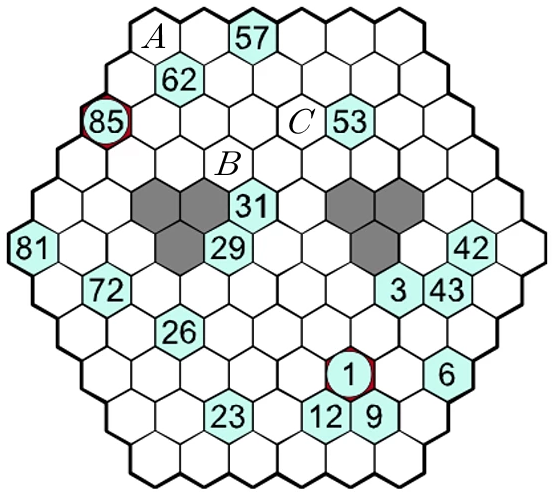# Beehive Hidato

Logic Level 2The picture above shows a Hidato puzzle. The aim of the puzzle is to fill each white/light blue cell with an integer between 1 and 85 (inclusive) so that each integer appears exactly once and consecutive integers appear in adjacent cells.

Let the number that takes place of the cell marked $A$ be denoted $A$, and so forth.

What is the value of $A+B+C$?

×